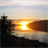# Python-Field Calculator Expression

2805
10
10-10-2014 07:00 AMNew Contributor III

I'm working on a model to create a field for Flood Risk.  What i'm needing to automate is that after the Flood Risk Field is added, i want to then calculate a risk of High, Moderate, or Low based on values from the Flood Risk Field using calculate field in modelbuilder.  So, I'm needing the statement to say something as listed below.  I'm fairly new to python and would appreciate any help for code to put into my field calculation.

If value in  Flood Zone is A,AE, AH, AO, etc then Flood Risk is High.

If value in Flood Zone is Whatever, then Flood Risk is Moderate.

If value in Flood Zone is X, then Flood Risk is Low.

FLD_ZONE                              FLD_RISK

A

AE

AH

AO

Whatever

X

Tags (4)
1 Solution

Accepted SolutionsbyMVP Esteemed Contributor

Although compact, some would argue the use of multiple compound statements isn't very pythonic.  PEP8 states compound statements are generally discouraged, especially with multi-clause statements.  Going with one-line statements may make the code longer, but it also improves readability, which makes debugging easier.  For me, I usually use the in operator for all conditional checks because it simplifies adding additional elements down the road if the data or criteria change.

```def CalcField(zone):
risk = ''
if zone in ('A', 'AE', 'AH', 'AO'):
risk = 'High'
elif zone in ('Whatever'):
risk = 'Moderate'
elif zone in ('X'):
risk = 'Low'
else:
risk = 'somedefaultvalue'
return risk

```
10 Repliesby
Regular Contributor III

I've never used Model Builder, preferring straight python, but here's a python function to do what you want:

```def CalcField(zone):
risk = ''
if zone in ('A', 'AE', 'AH', 'AO'): risk = 'High'
elif zone = 'Whatever': risk = 'Moderate'
elif zone = 'X': risk = 'Low'
else: risk = 'somedefaultvalue'
return risk

```

You want that last else in there to handle any cases that don't match the previous criteria, especially Null values. Call the function, passing in FLD_ZONE.

There is a CalculateField GP tool that I'd assume is available in Model Builder, but as I said, not familiar with MB, so not sure how that would integrate. Anyway, use what you can from the above.

Here's a good example from ESRI (3rd example, Calculate Ranges).byMVP Regular Contributor

Greg, you forgot the == comparison on the elif statements.

Jake, using Greg's updated Python code in the Calculate Field tool with Model Builder, complete the fields like this...

Field Name

FLD_RISK

Expression

```CalcField(!FLD_ZONE!)

```

Expression Type

PYTHON_9.3

Code Block

```def CalcField(zone):
risk = ''
if zone in ('A', 'AE', 'AH', 'AO'): risk = 'High'
elif zone == 'Whatever': risk = 'Moderate'
elif zone == 'X': risk = 'Low'
else: risk = 'somedefaultvalue'
return risk

```by
Regular Contributor III

Good catch Blake, thanks.byMVP Esteemed Contributor

Although compact, some would argue the use of multiple compound statements isn't very pythonic.  PEP8 states compound statements are generally discouraged, especially with multi-clause statements.  Going with one-line statements may make the code longer, but it also improves readability, which makes debugging easier.  For me, I usually use the in operator for all conditional checks because it simplifies adding additional elements down the road if the data or criteria change.

```def CalcField(zone):
risk = ''
if zone in ('A', 'AE', 'AH', 'AO'):
risk = 'High'
elif zone in ('Whatever'):
risk = 'Moderate'
elif zone in ('X'):
risk = 'Low'
else:
risk = 'somedefaultvalue'
return risk

```New Contributor III

Thanks all for the help.byMVP Esteemed Contributor

If I understand what you are saying correctly, the code could be updated to:  (Not sure if 0.2 is float or if you have '0.2 PCT' as text, I went with float)

```def CalcField(zone):
risk = ''
if zone in ('A', 'AE', 'AH', 'AO'):
risk = 'High'
elif zone in ('Whatever', 'Whatever2'):
risk = 'Moderate'
elif zone in ('X', 0.2):
risk = 'Low'
else:
risk = 'somedefaultvalue'
return risk
```byMVP Regular Contributor

Would it make sense to eliminate the assignment to the risk variable and just return the desired value instead?

```def CalcField(zone):
if zone in ('A', 'AE', 'AH', 'AO'):
return 'High'
elif zone in ('Whatever', 'Whatever2'):
return 'Moderate'
elif zone in ('X', 0.2):
return 'Low'
else:
return 'somedefaultvalue'

```byMVP Esteemed Contributor

Single vs. multiple exit points can be one of those discussions that turns into a holy war of sorts.  I do use multiple exit points at times in specific situations, but for this small block of code I liked the single exit point.  That said, others might like the way it reads with multiple exit points.byMVP Regular Contributor

Just came across this mention of returning values in a Python style guide and thought it would be relevant to post.

Code Style — The Hitchhiker's Guide to Python (Returning values)

It confirms what you said, Joshua: using one main exit point is preferred.